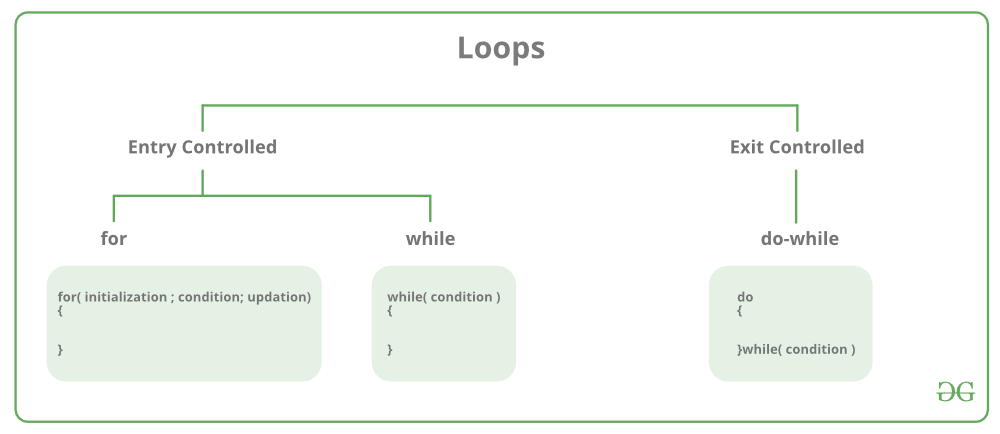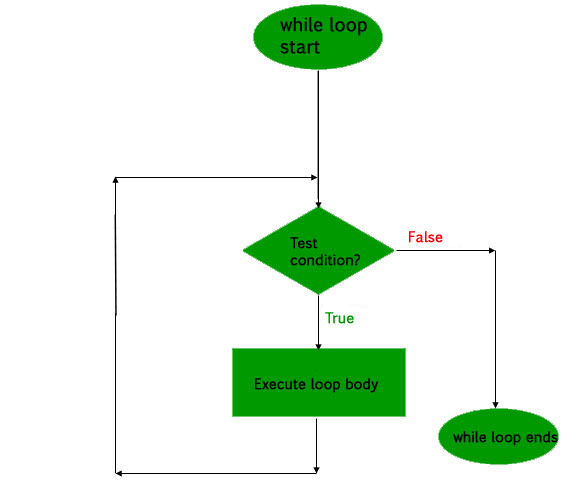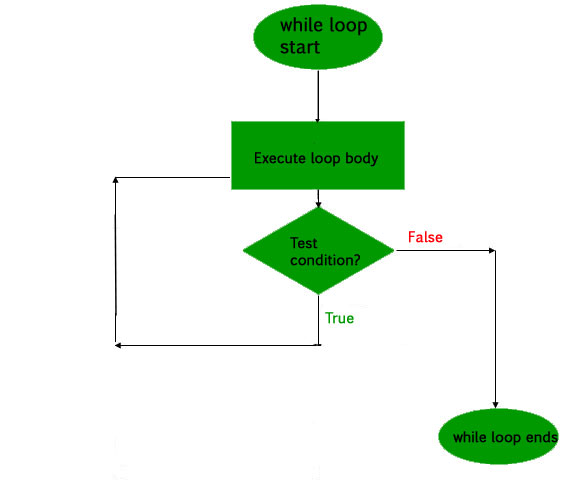# Loops in C and C++

• Difficulty Level : Easy
• Last Updated : 01 Jun, 2022

In programming, sometimes there is a need to perform some operation more than once or (say) n number of times. Loops come into use when we need to repeatedly execute a block of statements.

For example: Suppose we want to print “Hello World” 10 times. This can be done in two ways as shown below:

### Manual(general) Method (Iterative Method)

Manually we have to write the print() for C and cout for the C++ statement 10 times. Let’s say you have to write it 20 times (it would surely take more time to write 20 statements) now imagine you have to write it 100 times, it would be really hectic to re-write the same statement again and again. So, here loops have their role.

## C

 `// C program to illustrate need of loops` `#include `   `int` `main()` `{` `    ``printf``( ``"Hello World\n"``);` `    ``printf``( ``"Hello World\n"``);` `    ``printf``( ``"Hello World\n"``);` `    ``printf``( ``"Hello World\n"``);` `    ``printf``( ``"Hello World\n"``);` `    ``printf``( ``"Hello World\n"``);` `    ``printf``( ``"Hello World\n"``);` `    ``printf``( ``"Hello World\n"``);` `    ``printf``( ``"Hello World\n"``);` `    ``printf``( ``"Hello World\n"``);` `    `  `    ``return` `0;` `}`

## C++

 `// C++ program to illustrate need of loops` `#include ` `using` `namespace` `std;`   `int` `main()` `{` `    ``cout << ``"Hello World\n"``;` `    ``cout << ``"Hello World\n"``;` `    ``cout << ``"Hello World\n"``;` `    ``cout << ``"Hello World\n"``;` `    ``cout << ``"Hello World\n"``;` `    ``cout << ``"Hello World\n"``;` `    ``cout << ``"Hello World\n"``;` `    ``cout << ``"Hello World\n"``;` `    ``cout << ``"Hello World\n"``;` `    ``cout << ``"Hello World\n"``;` `    ``return` `0;` `}`

Output:

```Hello World
Hello World
Hello World
Hello World
Hello World
Hello World
Hello World
Hello World
Hello World
Hello World```

### Using Loops

In Loop, the statement needs to be written only once and the loop will be executed 10 times as shown below.  In computer programming, a loop is a sequence of instructions that is repeated until a certain condition is reached.

• An operation is done, such as getting an item of data and changing it, and then some condition is checked such as whether a counter has reached a prescribed number.
• Counter not Reached: If the counter has not reached the desired number, the next instruction in the sequence returns to the first instruction in the sequence and repeats it.
• Counter reached: If the condition has been reached, the next instruction “falls through” to the next sequential instruction or branches outside the loop.

There are mainly two types of loops:

1. Entry Controlled loops: In this type of loop, the test condition is tested before entering the loop body. For Loop and While Loop is entry-controlled loops.
2. Exit Controlled Loops: In this type of loop the test condition is tested or evaluated at the end of the loop body. Therefore, the loop body will execute at least once, irrespective of whether the test condition is true or false. the do-while loop is exit controlled loop.### for Loop

A for loop is a repetition control structure that allows us to write a loop that is executed a specific number of times. The loop enables us to perform n number of steps together in one line.
Syntax:

```for (initialization expr; test expr; update expr)
{
// body of the loop
// statements we want to execute
}```

Example:

```for(int i = 0; i < n; i++){
}```

In for loop, a loop variable is used to control the loop. First, initialize this loop variable to some value, then check whether this variable is less than or greater than the counter value. If the statement is true, then the loop body is executed and the loop variable gets updated. Steps are repeated till the exit condition comes.

• Initialization Expression: In this expression, we have to initialize the loop counter to some value. for example: int i=1;
• Test Expression: In this expression, we have to test the condition. If the condition evaluates to true then we will execute the body of the loop and go to update expression otherwise we will exit from the for a loop. For example: i <= 10;
• Update Expression: After executing the loop body this expression increments/decrements the loop variable by some value. for example: i++;

Equivalent Flow Diagram for loop:Example:

## C

 `// C program to illustrate for loop` `#include `   `int` `main()` `{` `    ``int` `i=0;` `    `  `    ``for` `(i = 1; i <= 10; i++)` `    ``{` `        ``printf``( ``"Hello World\n"``);    ` `    ``}`   `    ``return` `0;` `}`

## C++

 `// C++ program to illustrate for loop` `#include ` `using` `namespace` `std;`   `int` `main()` `{` `    ``for` `(``int` `i = 1; i <= 10; i++)` `    ``{` `        ``cout << ``"Hello World\n"``;` `    ``}`   `    ``return` `0;` `}`

Output:

```Hello World
Hello World
Hello World
Hello World
Hello World
Hello World
Hello World
Hello World
Hello World
Hello World```

### While Loop

While studying for loop we have seen that the number of iterations is known beforehand, i.e. the number of times the loop body is needed to be executed is known to us. while loops are used in situations where we do not know the exact number of iterations of the loop beforehand. The loop execution is terminated on the basis of the test conditions.
Syntax: We have already stated that a loop mainly consists of three statements – initialization expression, test expression, and update expression. The syntax of the three loops – For, while, and do while mainly differs in the placement of these three statements.

```initialization expression;
while (test_expression)
{
// statements

update_expression;
}```

Flow DiagramExample:

## C

 `// C program to illustrate while loop` `#include `   `int` `main()` `{` `    ``// initialization expression` `    ``int` `i = 1;`   `    ``// test expression` `    ``while` `(i < 6)` `    ``{` `        ``printf``( ``"Hello World\n"``);    `   `        ``// update expression` `        ``i++;` `    ``}`   `    ``return` `0;` `}`

## C++

 `// C++ program to illustrate while loop` `#include ` `using` `namespace` `std;`   `int` `main()` `{` `    ``// initialization expression` `    ``int` `i = 1;`   `    ``// test expression` `    ``while` `(i < 6)` `    ``{` `        ``cout << ``"Hello World\n"``;`   `        ``// update expression` `        ``i++;` `    ``}`   `    ``return` `0;` `}`

Output:

```Hello World
Hello World
Hello World
Hello World
Hello World```

### do-while loop

In do-while loops also the loop execution is terminated on the basis of test conditions. The main difference between a do-while loop and the while loop is in the do-while loop the condition is tested at the end of the loop body, i.e do-while loop is exit controlled whereas the other two loops are entry controlled loops.
Note: In a do-while loop, the loop body will execute at least once irrespective of the test condition.
Syntax

```initialization expression;
do
{
// statements

update_expression;
} while (test_expression);```

Note: Notice the semi – colon(“;”) in the end of loop.
Flow DiagramExample:

## C

 `// C program to illustrate do-while loop` `#include `   `int` `main()` `{` `    ``int` `i = 2; ``// Initialization expression`   `    ``do` `    ``{` `        ``// loop body` `        ``printf``( ``"Hello World\n"``);    `   `        ``// update expression` `        ``i++;`   `    ``}  ``while` `(i < 1);   ``// test expression`   `    ``return` `0;` `}`

## C++

 `// C++ program to illustrate do-while loop` `#include ` `using` `namespace` `std;`   `int` `main()` `{` `    ``int` `i = 2; ``// Initialization expression`   `    ``do` `    ``{` `        ``// loop body` `        ``cout << ``"Hello World\n"``;`   `        ``// update expression` `        ``i++;`   `    ``}  ``while` `(i < 1);   ``// test expression`   `    ``return` `0;` `}`

Output:

`Hello World`

In the above program, the test condition (i<1) evaluates to false. But still, as the loop is an exit – controlled the loop body will execute once.

### What about an Infinite Loop?

An infinite loop (sometimes called an endless loop ) is a piece of coding that lacks a functional exit so that it repeats indefinitely. An infinite loop occurs when a condition is always evaluated to be true. Usually, this is an error.
Using For loop:

## C

 `// C program to demonstrate infinite loops` `// using for and while` `// Uncomment the  sections to see the output`   `#include `   `int` `main ()` `{` `    ``int` `i;`   `    ``// This is an infinite for loop as the condition` `    ``// expression is blank` `    ``for` `( ; ; )` `    ``{` `       ``printf``(``"This loop will run forever.\n"``);` `    ``}`   `    ``// This is an infinite for loop as the condition` `    ``// given in while loop will keep repeating infinitely` `    ``/*` `    ``while (i != 0)` `    ``{` `        ``i-- ;` `        ``printf( "This loop will run forever.\n");` `    ``}` `    ``*/`   `    ``// This is an infinite for loop as the condition` `    ``// given in while loop is "true"` `    ``/*` `    ``while (true)` `    ``{` `        ``printf( "This loop will run forever.\n");` `    ``}` `    ``*/` `}`

## C++

 `// C++ program to demonstrate infinite loops` `// using for and while` `// Uncomment the  sections to see the output`   `#include ` `using` `namespace` `std;` `int` `main ()` `{` `    ``int` `i;`   `    ``// This is an infinite for loop as the condition` `    ``// expression is blank` `    ``for` `( ; ; )` `    ``{` `        ``cout << ``"This loop will run forever.\n"``;` `    ``}`   `    ``// This is an infinite for loop as the condition` `    ``// given in while loop will keep repeating infinitely` `    ``/*` `    ``while (i != 0)` `    ``{` `        ``i-- ;` `        ``cout << "This loop will run forever.\n";` `    ``}` `    ``*/`   `    ``// This is an infinite for loop as the condition` `    ``// given in while loop is "true"` `    ``/*` `    ``while (true)` `    ``{` `        ``cout << "This loop will run forever.\n";` `    ``}` `    ``*/` `}`

Output:

```This loop will run forever.
This loop will run forever.
................... ```

Using While loop:

## C

 `#include `   `int` `main() {`   `    ``while` `(1)` `        ``printf``(``"This loop will run forever.\n"``);` `    ``return` `0;` `}`

## C++

 `#include ` `using` `namespace` `std;`   `int` `main()` `{`   `    ``while` `(1)` `        ``cout << ``"This loop will run forever.\n"``;` `    ``return` `0;` `}`

Output:

```This loop will run forever.
This loop will run forever.
................... ```

Using Do-While loop:

## C

 `#include `   `int` `main() {`   `    ``do``{` `        ``printf``(``"This loop will run forever.\n"``);` `    ``}` `      ``while``(1);` `    ``return` `0;` `}`

## C++

 `#include ` `using` `namespace` `std;`   `int` `main() {` ` `  `    ``do``{` `        ``cout << ``"This loop will run forever.\n"``;` `    ``} ``while``(1);` ` `  `    ``return` `0;` `}`

Output:

```This loop will run forever.
This loop will run forever.
................... ```

Important Points:

• Use for loop when a number of iterations are known beforehand, i.e. the number of times the loop body is needed to be executed is known.
• Use while loops, where an exact number of iterations is not known but the loop termination condition, is known.
• Use do while loop if the code needs to be executed at least once like in Menu-driven programs

Related Articles: